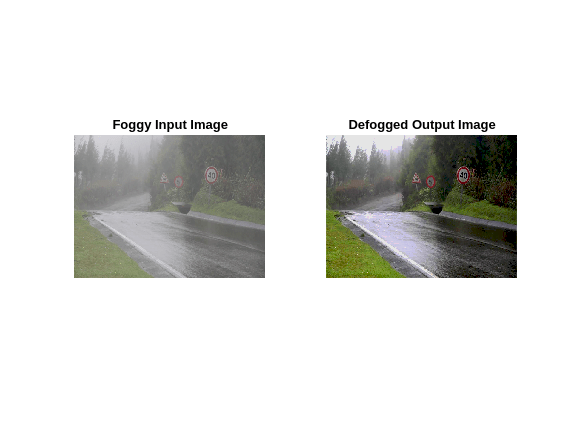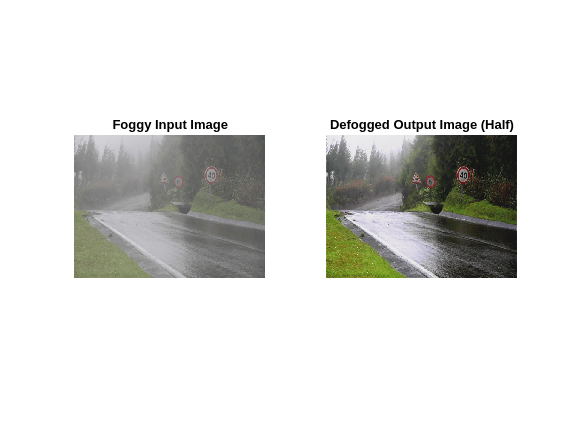# Fog Rectification

This example shows the use of image processing functions for GPU code generation. The example takes a foggy image as input and produces a defogged image. This example is a typical implementation of fog rectification algorithm. The example uses conv2, im2gray, and imhist functions.

### Third-Party Prerequisites

Required

This example generates CUDA® MEX and has the following third-party requirements.

• CUDA enabled NVIDIA® GPU and compatible driver. For half-precision code generation, the GPU must have a minimum compute capability of 6.0.

Optional

For non-MEX builds such as static, dynamic libraries or executables, this example has the following additional requirements.

### Verify GPU Environment

To verify that the compilers and libraries necessary for running this example are set up correctly, use the `coder.checkGpuInstall` function.

```envCfg = coder.gpuEnvConfig('host'); envCfg.BasicCodegen = 1; envCfg.Quiet = 1; coder.checkGpuInstall(envCfg);```

### The `fog_rectification` Entry-Point Function

The fog_rectification.m entry-point function takes a foggy image as input and returns a defogged image.

`type fog_rectification`
```function [out] = fog_rectification(input) %#codegen % % Copyright 2017-2023 The MathWorks, Inc. coder.gpu.kernelfun; % restoreOut is used to store the output of restoration restoreOut = zeros(size(input),"double"); % Changing the precision level of input image to double input = double(input)./255; %% Dark channel Estimation from input darkChannel = min(input,[],3); % diff_im is used as input and output variable for anisotropic % diffusion diff_im = 0.9*darkChannel; num_iter = 3; % 2D convolution mask for Anisotropic diffusion hN = [0.0625 0.1250 0.0625; 0.1250 0.2500 0.1250; 0.0625 0.1250 0.0625]; hN = double(hN); %% Refine dark channel using Anisotropic diffusion. for t = 1:num_iter diff_im = conv2(diff_im,hN,"same"); end %% Reduction with min diff_im = min(darkChannel,diff_im); diff_im = 0.6*diff_im ; %% Parallel element-wise math to compute % Restoration with inverse Koschmieder's law factor = 1.0./(1.0-(diff_im)); restoreOut(:,:,1) = (input(:,:,1)-diff_im).*factor; restoreOut(:,:,2) = (input(:,:,2)-diff_im).*factor; restoreOut(:,:,3) = (input(:,:,3)-diff_im).*factor; restoreOut = uint8(255.*restoreOut); %% % Stretching performs the histogram stretching of the image. % im is the input color image and p is cdf limit. % out is the contrast stretched image and cdf is the cumulative % prob. density function and T is the stretching function. % RGB to grayscale conversion im_gray = im2gray(restoreOut); [row,col] = size(im_gray); % histogram calculation [count,~] = imhist(im_gray); prob = count'/(row*col); % cumulative Sum calculation cdf = cumsum(prob(:)); % Utilize gpucoder.reduce to find less than particular probability. % This is equal to "i1 = length(find(cdf <= (p/100)));", but is % more GPU friendly. % lessThanP is the preprocess function that returns 1 if the input % value from cdf is less than the defined threshold and returns 0 % otherwise. gpucoder.reduce then sums up the returned values to get % the final count. i1 = gpucoder.reduce(cdf,@plus,"preprocess", @lessThanP); i2 = 255 - gpucoder.reduce(cdf,@plus,"preprocess", @greaterThanP); o1 = floor(255*.10); o2 = floor(255*.90); t1 = (o1/i1)*[0:i1]; t2 = (((o2-o1)/(i2-i1))*[i1+1:i2])-(((o2-o1)/(i2-i1))*i1)+o1; t3 = (((255-o2)/(255-i2))*[i2+1:255])-(((255-o2)/(255-i2))*i2)+o2; T = (floor([t1 t2 t3])); restoreOut(restoreOut == 0) = 1; u1 = (restoreOut(:,:,1)); u2 = (restoreOut(:,:,2)); u3 = (restoreOut(:,:,3)); % replacing the value from look up table out1 = T(u1); out2 = T(u2); out3 = T(u3); out = zeros([size(out1),3], "uint8"); out(:,:,1) = uint8(out1); out(:,:,2) = uint8(out2); out(:,:,3) = uint8(out3); end function out = lessThanP(input) p = 5/100; out = uint32(0); if input <= p out = uint32(1); end end function out = greaterThanP(input) p = 5/100; out = uint32(0); if input >= 1 - p out = uint32(1); end end ```

### Generate CUDA Code and MEX function

Set up the input for code generation and create a configuration for GPU code generation.

```inputImage = imread('foggyInput.png'); cfg = coder.gpuConfig('mex'); cfg.GpuConfig.EnableMemoryManager = true;```

### Run Code Generation

Generate the `fog_rectification_mex` MEX file by using the `codegen` command.

`codegen -args {inputImage} -config cfg fog_rectification`
```Code generation successful: View report ```

### Run the MEX Function with Foggy Image

Run the generated `fog_rectification_mex` with a foggy input image, and then plot the foggy and defogged images.

```[outputImage] = fog_rectification_mex(inputImage); % plot images p1 = subplot(1, 2, 1); p2 = subplot(1, 2, 2); imshow(inputImage, 'Parent', p1); imshow(outputImage, 'Parent', p2); title(p1, 'Foggy Input Image'); title(p2, 'Defogged Output Image');```Because of architectural differences between the CPU and GPU, numeric verification does not always match. This scenario is true when using the single data type or when performing integer type conversion in your MATLAB code. In this example, the integer type conversion in the `fog_rectification.m` entry-point function produces numeric differences with MATLAB simulation.

### Half-Precision

Computations in this example can also be done in half-precision floating point numbers, using the `fog_rectification_half_precision.m` entry-point function. To generate and execute code with half-precision data types, CUDA compute capability of 6.0 or higher is required. Set the `ComputeCapability` property of the code configuration object to `'6.0'`. For half-precision, the memory allocation (malloc) mode for generating CUDA code must be set to 'Discrete'.

```inputImageHalf = half(imread('foggyInput.png')); cfg = coder.gpuConfig('mex'); cfg.GpuConfig.EnableMemoryManager = true; cfg.GpuConfig.ComputeCapability = '6.0'; cfg.GpuConfig.MallocMode = 'Discrete'; codegen -args {inputImageHalf} -config cfg fog_rectification_half_precision```
```Code generation successful: View report ```

### Run the Half-Precision MEX Function with Foggy Image

Run the generated `fog_rectification_half_precision_mex` with a foggy input image, and then plot the foggy and defogged images.

```[outputImageHalf] = fog_rectification_half_precision_mex(inputImageHalf); % plot images p1 = subplot(1, 2, 1); p2 = subplot(1, 2, 2); imshow(inputImage, 'Parent', p1); imshow(outputImageHalf, 'Parent', p2); title(p1, 'Foggy Input Image'); title(p2, 'Defogged Output Image (Half)');```